Using the 3D Drawing Engine

We have provided quite a bit of background on how the 3D drawing engine works, and it's now time to delve into some deeper examples.

Interactive Rotating Cube

By now you should have a pretty good grasp on how transformations work, so you will revisit the cube example one last time and add a degree of interactivity. The FLA file that accompanies this example is drawing9.fla .

1. Create a new movie and set the window dimensions to 400x400. Select frame 1 on the timeline and add the following ActionScript code:

#include "draw3d.as"    // Initialize the 3d drawing object with 8 points and 6 faces    m = new Draw3D (8, 6);    // Define the point list    m.setPoint (0,  100,  100, -100); // Point 0    m.setPoint (1,  100, -100, -100); // Point 1    m.setPoint (2, -100, -100, -100); // Point 2    m.setPoint (3, -100,  100, -100); // Point 3    m.setPoint (4,  100,  100,  100); // Point 4    m.setPoint (5,  100, -100,  100); // Point 5    m.setPoint (6, -100, -100,  100); // Point 6    m.setPoint (7, -100,  100,  100); // Point 7    // Set the fill and line properties    m.setShadeOn ();    m.setLineWeight (2);    m.setLineColor (0, 0, 0);    m.setFillColor (.4, .4, .8);    // Define the faces    m.setFace (0, new Array (0, 1, 2, 3));    m.setFace (1, new Array (4, 7, 6, 5));    m.setFace (2, new Array (0, 4, 5, 1));    m.setFace (3, new Array (1, 5, 6, 2));    m.setFace (4, new Array (2, 6, 7, 3));    m.setFace (5, new Array (4, 0, 3, 7));    theta = 0;    m.scene.onEnterFrame = function () {     with (_root) {  mx = ((m.scene._xmouse) / 200);   my = ((m.scene._ymouse) / 200);  m.clearTransform();  m.rotateZ (theta);   m.rotateY ((-mx*45)*.0174);   m.rotateX (my*90*.0174);  m.applyPerspective (500);       theta += .02;       if (theta > 6.282) theta -= 6.282;       m.render ();     }    };
2. Finally, add a background image to taste, and test your movie (CTRL/CMD+ENTER).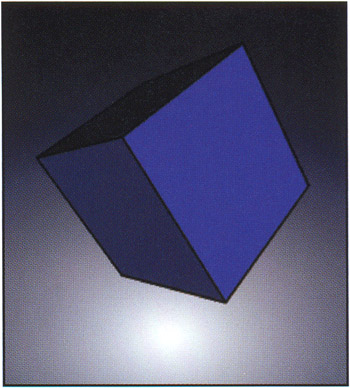3D Star

The cube is one of the classic 3D shapes, but it's only one of an infinite number of possible shapes that we can represent. In this example, you'll create a 3D star block (see drawing10.fla ).

1. A star is made up of ten points. If you were to plot a star on a piece of graph paper, you might come up with the following point assignments:2. Create a new movie with window dimensions 400x400, and then select frame 1 on the timeline and add the following ActionScript:

#include "draw3d.as"   m = new Draw3D (20, 12);  // Create the points we extracted from our graph paper drawing    // Top Star  m.setPoint (0, 6.5, 0, 4);   m.setPoint (1, 9, 5, 4);   m.setPoint (2, 14, 5.5, 4);   m.setPoint (3, 10.5, 9, 4);   m.setPoint (4, 12, 14, 4);   m.setPoint (5, 6.5, 11.5, 4);   m.setPoint (6, 2, 14, 4);   m.setPoint (7, 3.5, 9, 4);   m.setPoint (8, 0, 5.5, 4);   m.setPoint (9, 5, 5, 4);  // Bottom Star  m.setPoint (10, 6.5, 0, -4);   m.setPoint (11, 9, 5, -4);   m.setPoint (12, 14, 5.5, -4);   m.setPoint (13, 10.5, 9, -4);   m.setPoint (14, 12, 14, -4);   m.setPoint (15, 6.5, 11.5, -4);   m.setPoint (16, 2, 14, -4);   m.setPoint (17, 3.5, 9, -4);   m.setPoint (18, 0, 5.5, -4);   m.setPoint (19, 5, 5, -4);  // Set shading and color characteristics of the star  m. setShadeOn ();   m. setLineWeight (0);   m.setLineColor (1, .4, 0);   m.setFillColor (.1, .4, 1);   m.setFillAlpha (100);  // sides  for (i=0; i<9; i++) {   m.setFace (i, [i, i+1, 11+i, 10+i]);   }   m.setFace (9, [0, 9, 19, 10]);   theta = 0;   m.scene.onEnterFrame = function () {   with (_root) {   mx = ((m.scene._xmouse) / 200);   my = ((m.scene._ymouse) / 200);   m.clearTransform();   m.translate (-7, -7, 0);   m.scale (10, 10, 10);   m.rotateZ (theta);   m.rotateY ((-mx*45)*.0174);   m.rotateX (my*90*.0174);   m.applyPerspective (140);   theta += .04;   if (theta > 6.282) theta -= 6.282;   m.render ();]   }   };
3. Test your movie (CTRL/CMD+ENTER) and you should be seeing stars! Well, one star anyway.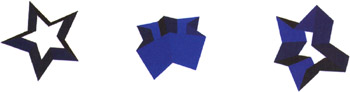Origami Swan

In the next example, you will render an origami swan. Origami is the Japanese art of folding paper, and origami artists have created some amazingly complex and beautiful works with only a single sheet of paper to work with. One popular figure that beginning origami students learn to make is the swan (remember to check out the swan in our example file drawing11.fla ).

1. You will begin by drawing the folded paper pieces that make up the swan on a sheet of graph paper. You can then use the grid to get the x- and y-coordinates of the polygonal pieces: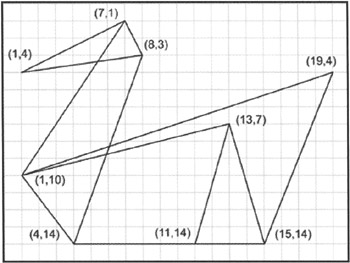We could then use a second piece of graph paper to plot a front view of the swan and then extract the z-coordinates of each piece. This particular model is fairly simple and we can probably guess approximately how far the points should extend from the x-y plane.

2. Open up a new movie and give it window dimensions of 500x300. Select frame 1 on the timeline and add the following script:

#include "draw3d.as"   m = new Draw3D (14, 10);  // Create the points we extracted from our graph paper drawing:  m.setPoint (0, 1, 4, 0);   m.setPoint (1, 7, 1, 0);   m.setPoint (2, 8, 3, 1);   m.setPoint (3, 8, 3, -1);   m.setPoint (4, 1, 10, 0);   m.setPoint (5, 4, 14, 2);   m.setPoint (6, 4, 14, -2);   m.setPoint (7, 13, 7, 5);   m.setPoint (8, 13, 7, -5);   m.setPoint (9, 11, 14, 8);   m.setPoint (10, 11, 14, -8);   m.setPoint (11, 15, 14, 6);   m.setPoint (12, 15, 14, -6);   m  .  setPoint(13, 19, 4, 0);  // Make the swan a translucent yellow with thin red lines  m.setShadeOn ();   m.setLineWeight (1);   m.setLineColor (1, .4, 0);   m.setFillColor (1, 1, 0);   m.setFillAlpha (70);  // Define the surfaces that make up the swan    // head  m.setFace (0, new Array(0, 1, 2));   m.setFace (1, new Array(0, 1, 3));  // neck  m.setFace (2, [4, 1, 2, 5]);   m.setFace (3, [4, 1, 3, 6]);  // outside wings  m.setFace (4, [5, 4, 7, 9]);   m.setFace (5, [6, 4, 8, 10]);  // inside wings  m.setFace (6, [5, 4, 7, 11]);   m.setFace (7, [6, 4, 8, 12]);  // body  m.setFace (8, [5, 4, 13, 11]);   m.setFace (9, [6, 4, 13, 12]);   theta = 0;  // Provide a 'show room floor' style rotation:  m.scene.onEnterFrame = function () {   with (_root) {   m.clearTransform();   m.scale (10, 10, 10);   m.rotateY (theta);   m.applyPerspective (500);   m.translate (50, -150, 0);   theta += .03;   if (theta > 6.282) theta -= 6.282;   m.render ();   }   };
3. As usual, press CTRL/CMD+ENTER to test the movie.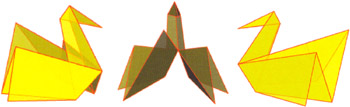There are actually a few subtle problems with this example. Many of the points that make up faces on the swan are not coplanar so, as the swan turns, if you examine the individual polygons that make up the swan you'll notice that some of them are not flat. When the 3D drawing engine renders them, it renders a polygonal shape that crosses itself (like the bow tie example at the beginning of the chapter). For the most part, this isn't a big problem itself. The technique we have chosen to draw nonplanar polygons is at least consistent. Shading them correctly is a bigger problem. Because the polygon isn't planar, there is no single angle between the surface and the light source that we can use for shading. There are also multiple interpretations of how such a surface should be shaded, if at all. The engine ignores this issue and uses the first three points in a polygon to form a flat surface to compute shading information.

Flying Rocket

In the previous examples, you created point and face information either from your head or with the help of some graph paper. More complex shapes are often harder to describe with these techniques. In this example we will show you a 3D modeling application that can be used to produce data you can render with your rendering engine. For this example, refer to the file drawing12.fla .

1. We began this exercise by creating a simple model of a spaceship in Blender, a free 3D modeling application. You can download the latest version of Blender from www.blender3d.org .2. Next up, we exported the model into Virtual Reality Modeling Language (VRML) format. The resulting file has the following structure:

#VRML V1.0 ascii    # Blender V2.0    Separator {    Switch {     DEF Body     Separator {       Coordinate3 {         point [              -2.213190 3.029455 0.314176,              -2.051762 0.365852 0.152740,             .             .             .              -0.386417 -0.200915 0.604752,              -0.722432 1.243950 0.940768,         ]       }       IndexedFaceSet {         coordIndex [              0, 1, 2, 3, -1,              4, 7, 6, 5, -1,             .             .             .              27, 31, 35, 39, -1,              28, 32, 35, 31, -1,         ]       }     }    }    }

This file is basically a list of points and a list of faces given in terms of the point indexes. Translating this file into something the drawing engine can use is a simple matter of cutting, pasting, and some minor editing. The first list of numbers in this example (abridged) represents points. Each point is space-separated and ends with a comma. The second list represents faces, and each face is represented with the indices into the point list that make up the face (the face ends with a -1 since this is not a valid index).

When designing characters , remember that Flash wasn't designed with 3D in mind. It's not optimized for 3D in the way that OpenGL or DirectX are, and it certainly can't compete with C, C++, or even Java in terms of computational speed. So keep everything simple!

3. Now we bring Flash into the procedure. Create a new movie and set the background color to black in the Property Inspector.

4. Click on frame 1 and then open the Actions panel. You will reuse all the classes you have created thus far. These are already available in the file named 3D.as , so you only need to include these classes using the #include action:

#include "draw3d.as"
5. There's a little bit of "hand waving" in this step you've taken a VRML file that was exported from the 3D modeler (Blender) and extracted the point and face lists. Each point entry in the VRML file corresponds to a Draw3D.setPoint () command and each face entry in the VRML file corresponds to Draw3D.setFace () . While you add the faces you also add some color and shading information:

m = new   Draw3D (29, 22);  // First enter the point list. The index is implicit    // in the VRML file; they are given in order.  m.setPoint (0, 2.532231, -2.585503, -14.208573);   m.setPoint (1, 1.097652, -1.116318, -12.507711);   m.setPoint (2, 1.366280, -1.391427, -10.272746);   m.setPoint (3, 2.361356, -2.410507, -11.191585);   m.setPoint (4, -2.536146, -2.538829, -14.208573);   m.setPoint (5, -1.090759, -1.080277, -12.507711);   m.setPoint (6, -1.361411, -1.353395, -10.272746);   m.setPoint (7, -2.363985, -2.365099, -11.191585);   m.setPoint (8, -0.000001, 3.614895, -14.208574);   m.setPoint (9, -0.000001, 1.561478, -12.507711);   m.setPoint (10, -0.000001, 1.945987, -10.272747);   m.setPoint (11, -0.000001, 3.370310, -11.191586);   m.setPoint (12, 2.195727, 2.195726, -3.261939);   m.setPoint (13, 3.105227, -0.000000, -3.261939);   m.setPoint (14, 2.195727, -2.195727, -3.261939);   m.setPoint (15, -0.000000, -3.105227, -3.261939);   m.setPoint (16, -2.195727, -2.195727, -3.261939);   m.setPoint (17, -3.105227, -0.000000, -3.261939);   m.setPoint (18, -2.195727, 2.195726, -3.261939);   m.setPoint (19, -0.000001, 3.105227, -3.261940);   m.setPoint (20, -0.000001, 1.414213, -12.454270);   m.setPoint (21, -1.000000, 0.999999, -12.454270);   m.setPoint (22, -1.414214, -0.000001, -12.454270);   m.setPoint (23, -1.000000, -1.000001, -12.454270);   m.setPoint (24, -0.000000, -1.414214, -12.454270);   m.setPoint (25, 1.000000, -1.000001, -12.454270);   m.setPoint (26, 1.414213, -0.000001, -12.454270);   m.setPoint (27, 1.000000, 0.999999, -12.454270);   m.setPoint (28, -0.032325, 0.007158, 4.384903);  // Set some properties for the base of the rocket.  m.setShadeOn ();   m.setLineWeight (0);   m.setFillColor (1, .2, .2);  // Begin entering faces that make up the rocket.  m.setFace (0, new Array (0, 3, 2, 1));   m.setFace (1, new Array (4, 7, 6, 5));   m.setFace (2, new Array (8, 11, 10, 9));   m.setFace (3, new Array (19, 12, 27, 20));   m.setFace (4, new Array (19, 20, 21, 18));   m.setFace (5, new Array (18, 21, 22, 17));   m.setFace (6, new Array (17, 22, 23, 16));   m.setFace (7, new Array (16, 23, 24, 15));   m.setFace (8, new Array (15, 24, 25, 14));   m.setFace (9, new Array (14, 25, 26, 13));   m.setFace (10, new Array (27, 12, 13, 26));   m.setFace (11, new Array (18, 28, 19));   m.setFace (12, new Array (12, 19, 28));   m.setFace (13, new Array (12, 28, 13));   m.setFace (14, new Array (17, 28, 18));   m.setFace (15, new Array (16, 28, 17));   m.setFace (16, new Array (13, 28, 14));   m.setFace (17, new Array (14, 28, 15));   m.setFace (18, new Array (15, 28, 16));  // The next three faces correspond to the back    // of the rocket. We will make them yellow.  m.setFillColor (1, 1, .2);   m.setFace (19, new Array (22, 25, 24, 23));   m.setFace (20, new Array (21, 26, 25, 22));   m.setFace (21, new Array (20, 27, 26, 21));
6. The final block of code defines the behavior of your rocket. We want this example to be a bit more attention- grabbing than our previous examples and have some interesting motion interactions. You will base the rocket's position and angle on the mouse position, but you will also add a gentle back-and-forth thrusting motion and a slight rotation to the rocket to make it seem like it's moving:

thrust = 0;   rocket_rotation = 0;   m.scene.onEnterFrame = function () {   with (_root) {  // Mouse coordinates scaled to be between -1 and 1  mx = ((m.scene._xmouse) / 200);   my = ((m.scene._ _ymouse) / 200);  // Reset all transformations  m.clearTransform();  // Scale the rocket a bit bigger  m.scale (15, 15, 15);  // Appy a subtle rotation to the rocket so it's always       // spinning  m.rotateZ (rocket__rotation*.0174) ;  // Orient the rocket in the right direction  m.rotateX (180*.0174);  // Use the mouse position to angle the rocket  m.rotateY ((180-mx*20)*.0174);   m.rotateX (my*45*.0174);  // In addition to a translation based on the       // mouse we add a little back and forth thrusting  m.translate (100*mx, 100*my, Math.abs(thrust-100));  // Set a moderate level of perspective  m.applyPerspective (500);  // Render the scene  m.render ();  // Update a few variables for the next iteration       thrust += 5;  if (thrust == 200) thrust = 0;   rocket_rotation += 5;   if (rocket_rotation == 360) rocket_rotation -= 360;   }   };
7. That's the end of the code. Now you just need to put the rocket into context. On a new layer, draw about 20 stars or so. Select all of the stars and then convert them to a movie clip by selecting Insert > Convert to Symbol.... Name this movie clip stars .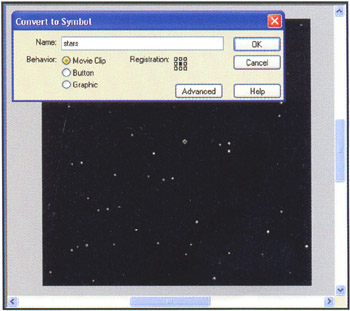8. Select the stars symbol and select Insert > Convert to Symbol... again. Name this symbol star_field .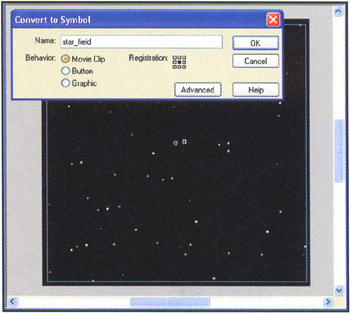9. Double-click the star_field symbol to begin editing it. Create a new layer and copy the frame in the first layer to the second layer. Add keyframes to frame 30 on both layers .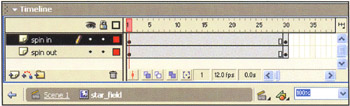10. Now go to frame 1 and select the movie clip on the top layer. Open the Property Inspector and set the alpha to 4%. In the Transform window (CTRL/CMD+T), set the rotation to 45 degrees and the x and y scaling to 60%.

11. Next, go to frame 30. Select the symbol on the bottom layer. Open the Property Inspector and set the alpha here to 4%. In the Transform window, set the rotation to -45 degrees and the x and y scaling to 160%.

12. Finally, click on frame 1 of each layer and then select Insert > Create Motion Tween (or use the Property Inspector).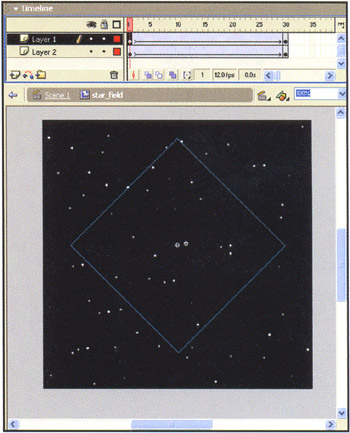13. If you test the movie now, the rocket should seem to be spinning into space while the mouse steers it.Flash 3D Cheats Most Wanted
ISBN: 1590592212
EAN: N/A
Year: 2002
Pages: 97